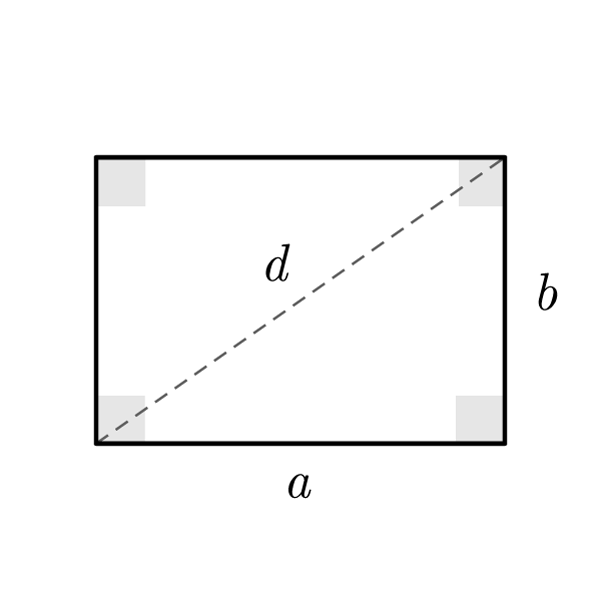# Rectangle

 Length $$a$$ Width $$b$$ Diagonal $$d$$
$$2p = 2a+2b$$
Perimeter
$$A = a \times b$$
Area
$$a = \frac{A}{b}$$
Length
$$b = \frac{A}{a}$$
Width
$$d = \sqrt{{a}^{2} + {b}^{2}}$$
Diagonal (Pythagoras' theorem)
$$a = \sqrt{{d}^{2} - {b}^{2}}$$
Length
$$b = \sqrt{{d}^{2} - {a}^{2}}$$
Width

## Definition

A rectangle is a quadrilateral with internal angles congruent (right angles) and opposite sides congruent.

## Properties

1. One side is larger (length) and the other smaller (width). The opposite sides are congruent (the sides are congruent in group of two)
2. The sides can be also written as base (length) and height (width)
3. Four congruent right angles## Rectangle Formulas

Data Formula
Perimeter 2p = 2 × a + 2 × b
Area A = a × b
Diagonal d = √( a2 + b2 )
Length a = A / b
Width b = A / a
Length a = √( d2 - b2 )
Width b = √( d2 - a2 )